# Arithmetic progression

In some AP applies:

5a2 + 7a5 = 90
s3 = 12

Find the first member a =? and difference d = ?

a =  2
d =  2

### Step-by-step explanation:

5•(a+d)+7•(a+4•d) = 90
a+(a+d)+(a+2•d) = 12

12a+33d = 90
3a+3d = 12

a = 2
d = 2

Our linear equations calculator calculates it.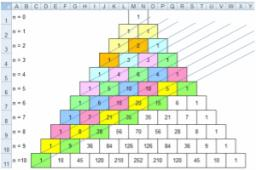Did you find an error or inaccuracy? Feel free to write us. Thank you!Tips to related online calculators
Do you have a linear equation or system of equations and looking for its solution? Or do you have a quadratic equation?

## Related math problems and questions:

• Fifth memberDetermine the fifth member of the arithmetic progression, if the sum of the second and fifth members equal to 73, and difference d = 7.
• Difference AP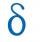Calculate the difference of arithmetic progression if the sum of its first 19 members Sn = 8075 and the first member is a1 = 20
• Geometric seqFind the third member of geometric progression if a1 + a2 = 36 and a1 + a3 = 90. Calculate its quotient.
• Determine AP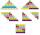Determine the difference of the arithmetic progression if a3 = 7, and a4 + a5 = 71
• Difference AP 4Calculate the difference of the AP if a1 = 0.5, a2 + a3 = -1.1
• Sequences AP + GPThe three numbers that make up the arithmetic sequence have the sum of 30. If we subtract from the first 5, from the second 4 and keep the third, we get the geometric sequence. Find AP and GP members.
• Third memberDetermine the third member of the AP if a4=93, d=7.5.
• Geometric progressionIn geometric progression, a1 = 7, q = 5. Find the condition for n to sum first n members is sn≤217.
• Find the 13Find the equation of the circle inscribed in the rhombus ABCD where A[1, -2], B[8, -3] and C[9, 4].
• Arithmetic progression 2The 3rd term of an Arithmetic progression is ten more than the first term, while the fifth term is 15 more than the second term. Find the sum of the 8th and 15th terms of the Arithmetic progression if the 7th term is seven times the first term.
• AP - simpleFind the first ten members of the sequence if a11 = 132, d = 3.
• Four swords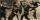Obelix has three helmets, four swords, and five shields. How many words must make at the blacksmith forge Metallurgix to be able to walk another 90 days in unique armor?
• AP 6Calculate the first five items of an arithmetic sequence if it is given: a2 – a3 + a5 = 20 a1 + a6 = 38
• AP - basicsDetermine first member and differentiate of the the following sequence: a3-a5=24 a4-2a5=61
• In aIn a triangle, the aspect ratio a: c is 3: 2, and a: b is 5: 4. The perimeter of the triangle is 74cm. Calculate the lengths of the individual sides.
• Insert 3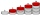Insert five arithmetic progression members between -7 and 3/2.
• Statistics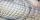The sum of all deviations from the arithmetic mean of the numerical sequence 4, 6, 51, 77, 90, 93, 95, 109, 113, 117 is: